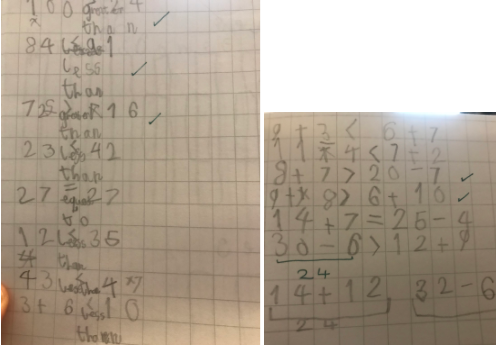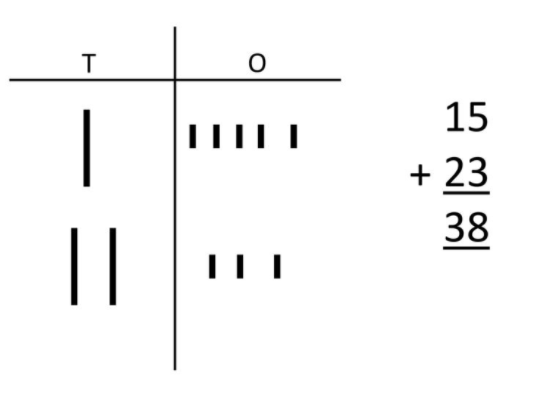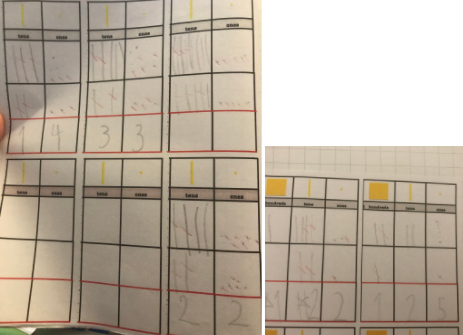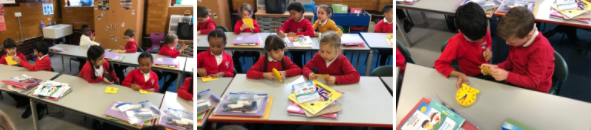••••••••# Maths

#### Summer 1

This week we have been learning about 3D shapes. We have learnt about the properties of 3D shapes.

Properties of 3D Shapes

If you would like to view this content please

We have also printed the faces of 3D shapes.We used marshmallows and spaghetti to make 3D shapes. We were able to discuss their vertices and edges.This week we learnt about place value.Why don't you practise your hundreds, tens and units understanding by playing these games?Place Value PartitioningWe have been adding and subtracting 1, 10 and 100 from numbers.Why don't you practise with these games?

Adding and Subtracting 1, 2, 3, 4, 5Adding and Subtracting 1 and 10This week we have been learning about mass and weight.

We ordered objects based on their weights and chose which weight was suitable for each object.We then used weights to estimate the weight of objects and used a scale to check our estimates.Why don't you practise reading different types of scales with these games?

Balance ScalesDigital ScalesThis week we have been remembering how to solve addition word problems.We have also been ordering the liquids in bottles.We estimated whether the contents were more or less that 1 litre and measured it using measuring jugs.After that we worked out scales and used this to read the contents of  measuring jugs.#### Autumn 1

This term, we have been investigating the hundred square and its structure.We have been learning about place value and have created numbers using bead strings, numicon and dienes.We have also drawn numbers using dienes.We have also been learning how to find one and ten more or less than a number.We have been practising our ten times tables.

If you would like to view this content please

We have been comparing numbers using the greater than, less than and equal to signs and have also ordered numbers.This week we are learning to add dienes.We have been learning how to subtract using dienes. It is important to neatly cross out the tens and ones as we subtract them. What is left is the answer.We have been learning how to tell the time using o'clock, half past, quarter to and quarter past. We are trying to practise this every day.